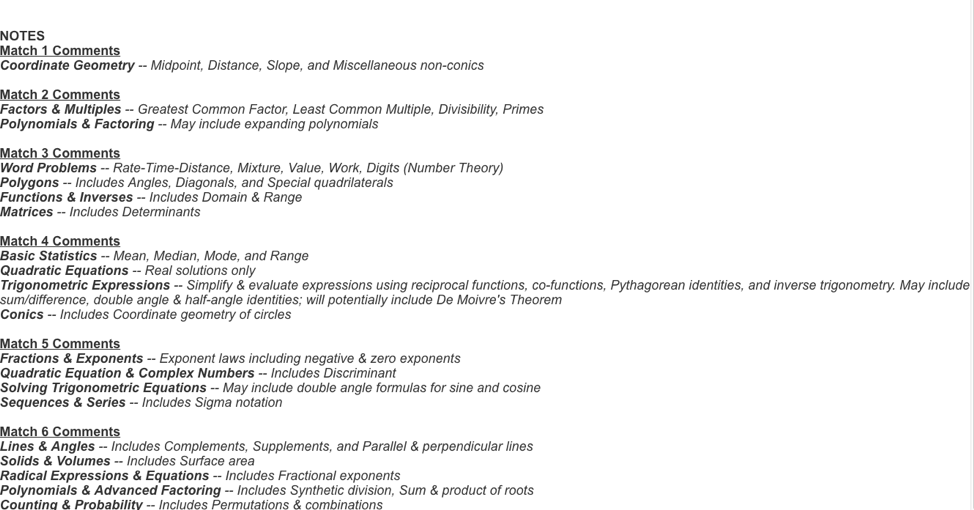# Match Topics

Match 1

Round 1: Arithmetic & Basic Topics: Percentages

Round 2: Algebra I: Solving Equations

Round 3: Geometry: Triangles and Quadrilaterals

Round 4: Algebra II: Systems of Equations

Round 5: Trig & Advanced Topics: Right Triangles

Round 6: Coord Geometry & Discrete Math: Coordinate Geometry

Match 2

Round 1: Arithmetic & Basic Topics: Factors and Multiples

Round 2: Algebra I: Polynomials and Factoring

Round 3: Geometry: Area and Perimeter

Round 4: Algebra II: Inequalities and Absolute Value

Round 5: Trig & Advanced Topics: Laws of Sines and Cosines

Round 6: Coord Geom & Discrete Math: Equations of Lines

Match 3

Round 1: Arithmetic & Basic Topics: Bases and Scientific Notation

Round 2: Algebra I: Word Problems

Round 3: Geometry: Polygons

Round 4: Algebra II: Functions and Inverses

Round 5: Trig & Advanced Topics: Exponential and Logarithmic Expressions and Equations

Round 6: Coord Geom & Discrete Math: Matrices

Match 4

Round 1: Arithmetic & Basic Topics: Basic Statistics

Round 2: Algebra I: Quadratic Equations

Round 3: Geometry: Similarity

Round 4: Algebra II: Variation

Round 5: Trig & Advanced Topics: Trig Expressions and De Moivre’s Theorem

Round 6: Coord Geom & Discrete Math: Conic Sections

Match 5

Round 1: Arithmetic & Basic Topics: Fractions and Exponents

Round 2: Algebra I: Fractional Expressions and Equations

Round 3: Geometry: Circles

Round 4: Algebra II: Quadratic Equations and Complex Numbers

Round 5: Trig & Advanced Topics: Solving Trig Equations

Round 6: Coord Geom & Discrete Math: Sequences and Series

Match 6

Round 1: Arithmetic & Basic Topics: Lines and Angles

Round 2: Algebra I: Literal Equations

Round 3: Geometry: Solids and Volume

Round 4: Algebra II: Radical Expressions and Equations